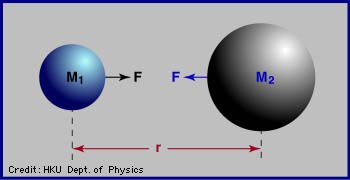﻿ Newton's Laws
 Kepler did not know the reasons behind his three laws. He just deduced them from the observation data. Issac Newton, the founder of modern physics, provided the theoretical basis, which means he deduced the three laws, and more, from less assumptions.According to Newton's theory, there is an attractive force between any two massive bodies. If the masses of the two bodies are M1 and M2 and the distance between them is r, then the attractive force F is equal to F = G M1 M2 / r2 where G is the gravitational constant, which is a very small number. The product of M1 x M2 is then divided by the square of the distance between the two bodies.
 This attractive force makes it understandable why the Moon ( M1 ) orbits around the Earth ( M2 ). If the Earth was not there, the Moon would travel in a straight line, that is, fly away. The Earth and the Moon attract each other, so the Moon 'falls' to the Earth, just like a ball does. This falling keeps the Moon in its orbit. Similarly, the Earth and other planets 'fall' to the Sun constantly. Newton's gravitational theory also predicts that in general, the orbit of an object can be any of the four conic sections: circle, ellipse, parabola and hyperbola; as well as the straight line. Conic sections get their names because they take the shapes of the cross sections of a cone. Astronomers have found some comets in parabolic or hyperbolic orbits.